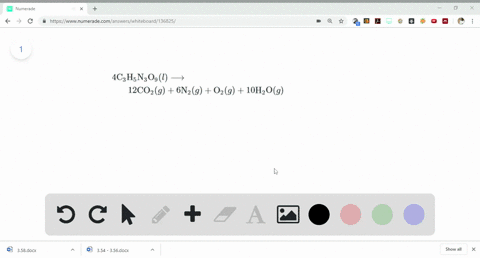Sign up for our free STEM online summer camps starting June 1st!View Summer Courses### Detonation of nitroglycerin proceeds as follows: …

06:52University of New Mexico

Need more help? Fill out this quick form to get professional live tutoring.

Get live tutoring
Problem 69

A piece of aluminum foil 1.00 $\mathrm{cm}^{2}$ and 0.550 -mm thick is allowed to react with bromine to form aluminum bromide.
(a) How many moles of aluminum were used? (The density of aluminum is 2.699 $\mathrm{g} / \mathrm{cm}^{3} .$ ) (b) How many grams of aluminum bromide form, assuming the aluminum reacts completely?

a) 0.0550 mol Al
b) 1.47 $\mathrm{g}$

## Discussion

You must be signed in to discuss.

## Video Transcript

for discussion. Firstly, we need to deter mined the volume off aluminum for we know square is one centimetre thickness along with zero point 550 The volume turns out to be 0.55 centimeter square. We know mask is equal to volume multiplied with density. Doing that, we have the total mass. Now we need to calculate the number off moles off aluminum. So, Molly grimace off. Aluminum is Tony six point I need you can round it off to 27 hence 0.148 grams off aluminum will have 0.550 into Denver's Tobar minus three moves for part B. We need to balance the chemical equation between aluminum on dhe Romi. So to aluminum plus three. Beer, too, will provide toe a l br three. No equation is satisfied. Now we need to find the mole a mask off L. B R. Three that turns out to be 26.98 for aluminum last three. Adam's off Ramin having more legal Omar's off 79.90 which gives us a tool marks off to 66.69 grams for more. Now we know that mosques equals Move into Moeller muss, which is 5.5 into 10 days, four minus three multiplied with 266 point 69 rooms.